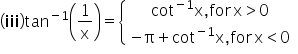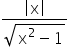5 You must Know things about today Reach me for Website Development, Mobile App Development, Digital Marketing and SEO services. Hidden things of Indian Politicians and Extreme research of Topics

# Theory Part 2

Property II:

(i) sin (sin-1 x) = x= cos (cos-1 x),                for all x ∈ [-1, 1]

Property III:

(i) sin-1 (-x) = -sin-1 (x),                  for all x ∈ [-1, 1]

(ii) cos-1 (-x) = π - cos-1 (x),           for all x ∈ [-1, 1]

(iii) tan-1 (-x) = -tan-1 x                   for all x ∈ R

(iv) cosec-1 (-x) = -cosec-1 x,         for all x ∈ [-∞, -1] υ [1, ∞)

(v) sec-1 (-x) = π - sec-1 x,              for all x ∈ [-∞, -1] υ [1, ∞)

(vi) cot-1 (-x) = π - cot-1 x,              for all x ∈ R

Property IV:

(i) sin-1 x + cos-1 x = (π/2),            for all x ∈ [-1, 1]

(ii) tan-1 x + cot-1 x = (π/2),           for all x ∈ R

(iii) sec-1 x + cosec-1 x = (π/2),      for all x ∈ (-∞, -1] υ [1, ∞)

Property V:

(i) sin-1 (1/x) = cosec-1 x,               for all x ∈ (-∞, -1] υ [1, ∞)

(ii) cos-1 (1/x) = sec-1 x,                 for all x ∈ (-∞, -1] υ [1, ∞)Property VI:

(i) sin-1 x = cos-1 (1 – x2)1/2 = tan-1 [x/(1 – x2)1/2]

= cot-1 [((1 – x2)1/2)/x] = sec-1 [1/(1 – x2)1/2] = cosec-1 (1/x), x ∈ (0, 1)

(ii) cos-1 x = sin-1 (1 – x2)1/2 = tan-1 [((1 – x2)1/2)/x]

= cot-1 [x/(1 – x2)1/2] = sec-1 (1/x) = cosec-1 [1/(1 – x2)1/2], x ∈ (0, 1)

(iii) tan-1 x = sin-1 [x/(1 + x2)1/2] = cos-1 [1/(1 + x2)1/2]

= cot-1 (1/x) = sec-1 [(1 + x2)1/2] = cosec-1 [((1 + x2)1/2)/x], x > 0

Property VII:

(i) sin (cos-1 x) = cos (sin-1 x) = (1 – x2)1/2, -1 ≤ x ≤ 1.

(ii) tan (cot-1 x) = cot (tan-1 x) = (1/x), x ∈ R, x ≠ 0.

(iii) cosec (sec-1 x) = sec (cosec-1 x) =. |x| > 1.

Related Keywords